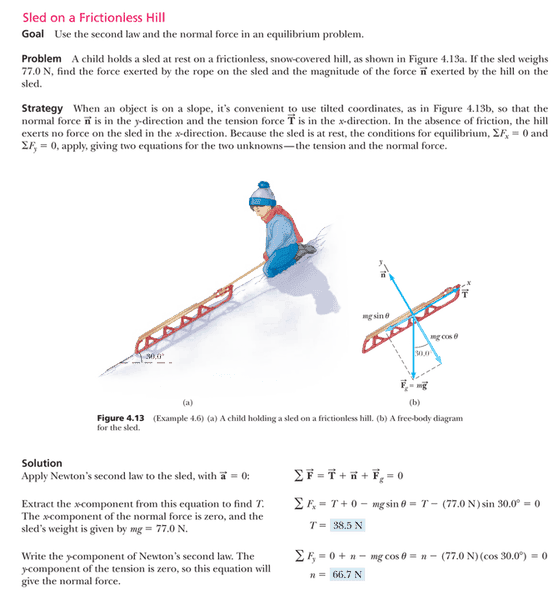# When to use cos or sin in physics problems

• Raza

#### Raza

Hi, I get really confused when to use the cos function or the sin function. for example, I was looking at this yesterday and thought the other way around of the correct answer. Why did it chose sin function for tension and cos function for the normal force?This problem doesn't explain why it chose the trigometric function for each question.

Thank You.

The easy way is to think what happens as the angle goes to zero. As angle->0 sin -> 0 cos->1

In your example, the force along the slope will go to zero as it gets horizontal (so is sin) the force on the runners will increase, until it is the whole weight (so is cos)

Whatever function you choose depends on the components of the vectors that you need.

For instance in your problem, you need the gravity component along the slope from a vertical force. This will resolve itself into a normal force that you can easily relate to the angle of the slope itself with the cos function. (Refer to the diagram.)

As pointed out already this then would be the adjacent side to the angle.

In figuring out the acceleration along the slope then you need the other component of the vector down the slope. Since it is the side opposite the angle then sine is what you need to use.